#### You may also like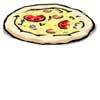### Pizza Portions

My friends and I love pizza. Can you help us share these pizzas equally?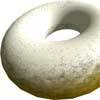### Doughnut

How can you cut a doughnut into 8 equal pieces with only three cuts of a knife?### Pies

Grandma found her pie balanced on the scale with two weights and a quarter of a pie. So how heavy was each pie?

# Extending Fraction Bars

##### Age 7 to 11Challenge Level

This activity follows on from More Fraction Bars.

Look at these different coloured bars: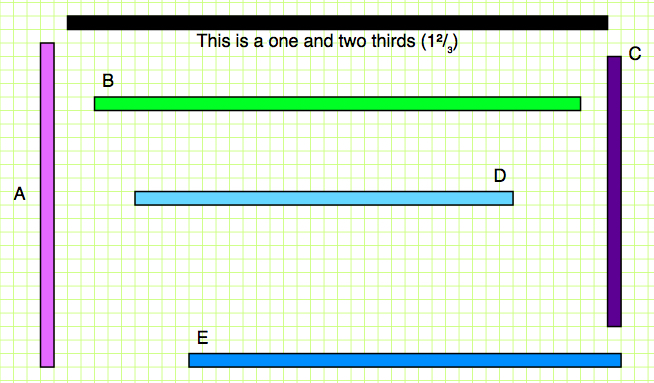You might like to download a printable version of them here .

Put the bars in size order - can you do it without cutting them out?

Now focus on this bar: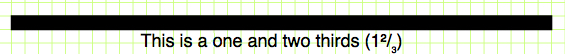This bar represents one whole and two thirds, or the number 1$\frac{2}{3}$. You might find it easier to think of it as a bar which is 'one whole' that has been stuck to a bar two-thirds the size of it.

We are thinking about all the other coloured bars as fractions of this bar, so we are thinking about them as fractions of 1$\frac{2}{3}$.

For example, look at Bar A below: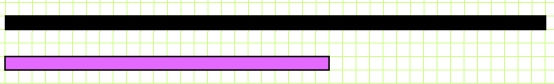Drawing lines helps us measure it against the black bar: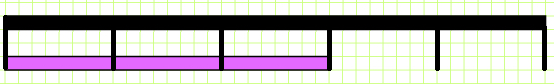What fraction of the black bar is Bar A?

Go through each of the other coloured bars and compare them to the black bar. What fraction of 1$\frac{2}{3}$ is each bar?

Write down your ideas for each bar. For example, you could write:
Bar A is three fifths of the black bar.
or
Bar A represents $\frac{3}{5}$ of 1$\frac{2}{3}$.
or
Bar A represents a whole.

Can you work out how we came up with these three ideas?

Can you find different ideas for what fraction of 1$\frac{2}{3}$ each bar represents?